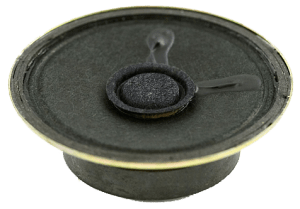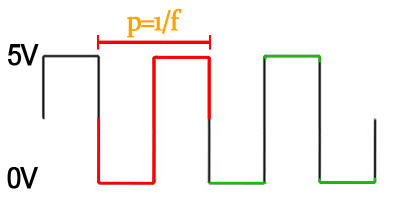# Driving 5 Speakers Simultaneously with an Arduino

This post was featured on the Hack-a-Day Blog on 9/14/10
Mimi Yin has a neat implementation of this code for an interactive sculpture.I’m working on a project where I want to drive 5 speakers independently – each with a variable volume and set frequency (though the frequency of each speaker is different).  I examined several methods for generating the 5 square waves in hardware – using a 555 timer, oscillating RC with schmitt trigger, and a bunch of opamp circuits.  Unfortunately, none of these generated tones as nice as the ones I got when simply using the arduino tone library.  The built-in tone() function allows you to generate a square wave with 50% duty cycle of your selected frequency on any pin on the arduino.  It relies on one of the arduino’s 3 timers to work in the background.  Specifically, it uses timer2, which is also responsible for controlling PWM on pins 3 and 11.  So you naturally loose that ability when using the tone() function.  But I wanted to make 5 tones simultaneously!  How to do it?

While the tone() function can only be run one instance at a time, I discovered (after much research), that I could hijack one of the timers (again, I chose timer2), and use some clever math and software interrupts to generate 5 tones simultaneously! I wanted to generate 5 tones of the following frequencies:

1. C = 131 Hz
2. D = 147 Hz
3. E = 165 Hz
4. G = 196 Hz
5. A = 220 Hz

Any music lovers out there will surely recognize this as the pentatonic scale (5 notes that nearly always sound good together). Now, think back to physics, and recall that period = 1/frequency.  In other words, each frequency pin will have to cycle from 0V to 5V and back again in the time dictated by the period for that note.  See the following picture:The red portion of the square wave represents one cycle.  From the formula above, we can determine the following periods required to generate each note:

1. C = 1/131 Hz = 7.6ms
2. D = 1/147 Hz = 6.8ms
3. E = 1/165 Hz = 6.0ms
4. G = 1/196 Hz = 5.1ms
5. A = 1/220 Hz = 4.5ms

But, remember that we are dealing with a microcontroller, so we need to flip the value twice every period.  If you take a look at the green portions on the image above you’ll see what I’m talking about.  In one red portion of the wave, the value needs to change twice.  So in reality, we actually need to be able to flip bits twice as fast as the times outlined above.  Dividing those values by two, we get the following:

1. C = 7.6ms/2 = 3.80ms
2. D = 6.8ms/2 = 3.40ms
3. E = 6.0ms/2 = 3.00ms
4. G = 5.1ms/2 = 2.55ms
5. A = 4.5ms/2 = 2.25ms

So, if we want to generate all these tones at the same time, we’re going to need to have an interrupt service that can activate at a Greatest Common Factor for all these values.  This interrupt request will toggle the appropriate pin every prescribed number of milliseconds, and we’ll get the tones we need! So, the least common multiple for the variables above would be: .05ms, or 50μs.  If we use this ideal value, we can construct an interrupt service request that will initiate every 50μs during our program.  Using some counters, we can flip the bits after the appropriate intervals!  Let’s calculate those counters now:

1. C = 3.80ms/50μs = 76 counts
2. D = 3.40ms/50μs = 68 counts
3. E = 3.00ms/50μs = 60 counts
4. G = 2.55ms/50μs = 51 counts
5. A = 2.25ms/50μs = 45 counts

In an ideal world, we would simply have a software interrupt trigger every 50μs, increment 5 separate counters each time, and toggle the associated pin when we reached the right value.  For example, every time counter1 reached 76, we would reset it to 0, and toggle the value of that pin.  If the only thing you were doing with your CPU was making noises, then this would work quite well.  But the whole reason for doing this in an interrupt routine is so that we can do other things simultaneously.  I had to trade off some frequency accuracy for a reduction in how often I activated the interrupt.  After some trial and error, I deemed the golden value to be 64μs.  It’s a little more than the ideal lowest common denominator, but still close enough that the notes sound good.  Using this new value, I calculated new counts, and implemented them in my interrupt routine:

1. C = 3.80ms/64μs ≈ 60 counts
2. D = 3.40ms/64μs ≈ 53 counts
3. E = 3.00ms/64μs ≈ 47 counts
4. G = 2.55ms/64μs ≈ 40 counts
5. A = 2.25ms/64μs ≈ 35 counts

And that’s it!  Using these values, I can simultaneously generate all five notes, while maintaining enough time to quickly update my LED Matrix.  Below is the final arduino code for making this work. Thanks to Sebastian Wallin for some awesome information on interfacing with arduino timer interrupts.

 //** Arduino – Drive Multiple Speakers with Interrupts **// //** http://www.jeremyblum.com/2010/09/05/driving-5-speakers-simultaneously-with-an-arduino/ **// /* Timer reload value, globally available */ unsigned int tcnt2; /* Toggle HIGH or LOW digital write */ int toggle1 = 0; int toggle2 = 0; int toggle3 = 0; int toggle4 = 0; int toggle5 = 0; /* Keep track of when each note needs to be switched */ int count1 = 0; int count2 = 0; int count3 = 0; int count4 = 0; int count5 = 0; /* Frequency Output Pins */ #define FREQ1 9 #define FREQ2 10 #define FREQ3 11 #define FREQ4 12 #define FREQ5 13 //Setup Function will run once at initialization void setup() { /* First disable the timer overflow interrupt*/ TIMSK2 &= ~(1< 8us. * (desired period) / 8us = 8. * MAX(uint8) – 8 = 248; */ /* Save value globally for later reload in ISR */ tcnt2 = 248; /* Finally load end enable the timer */ TCNT2 = tcnt2; TIMSK2 |= (1<

view raw
multi-speaker.ino
hosted with ❤ by GitHub